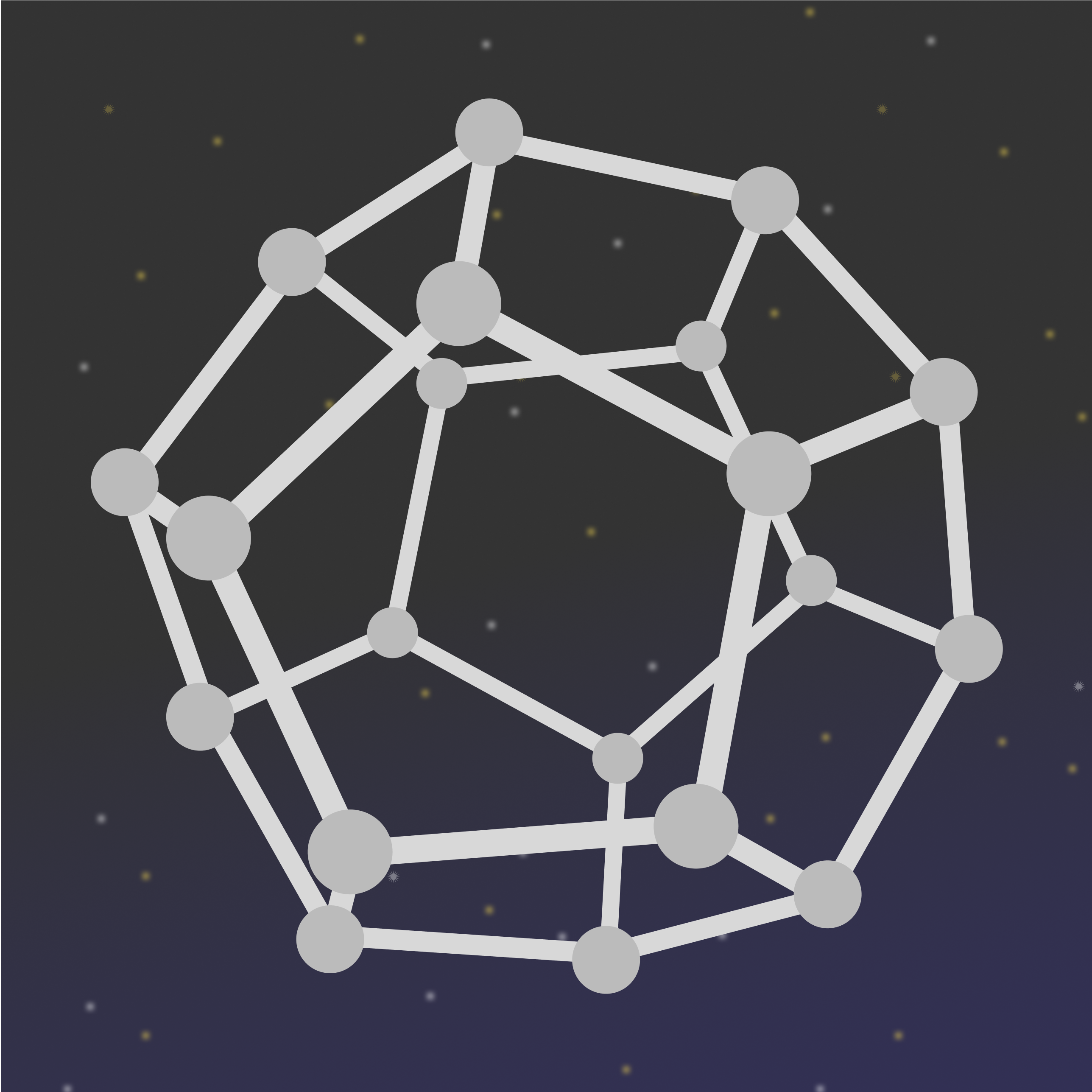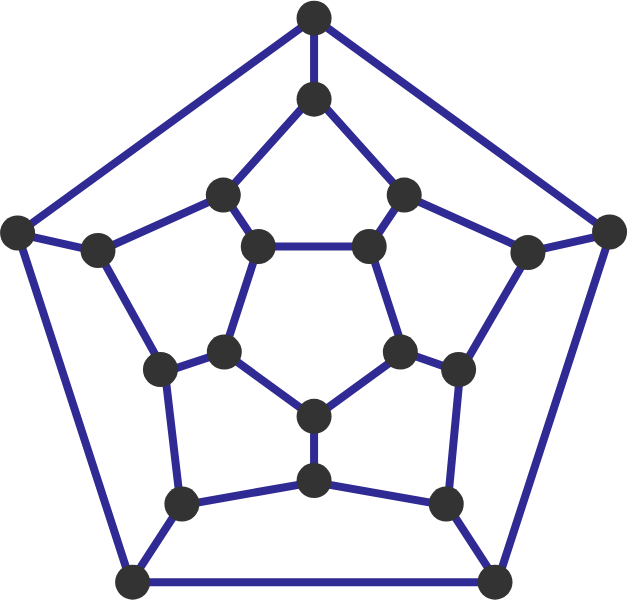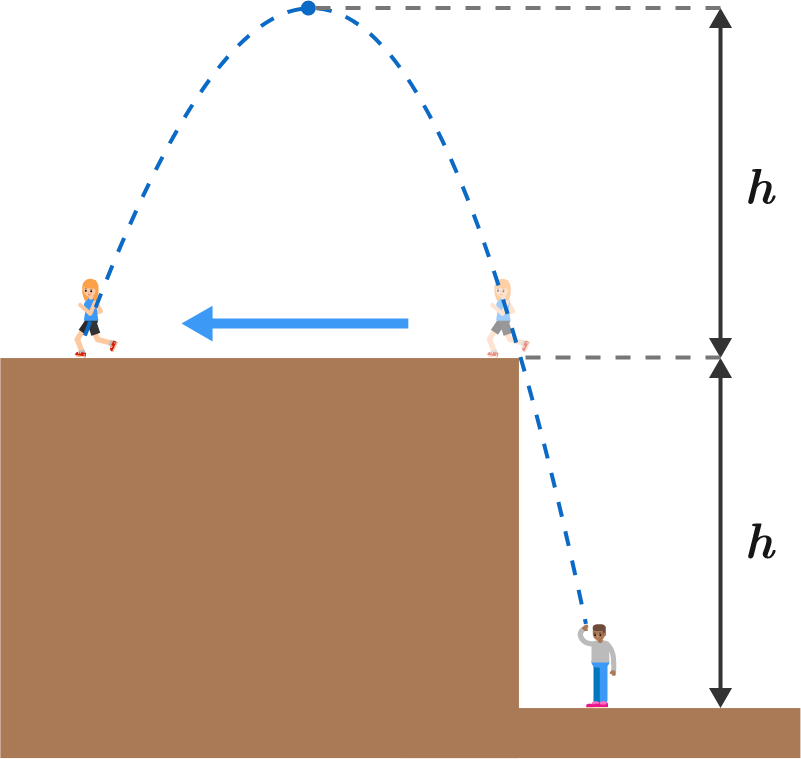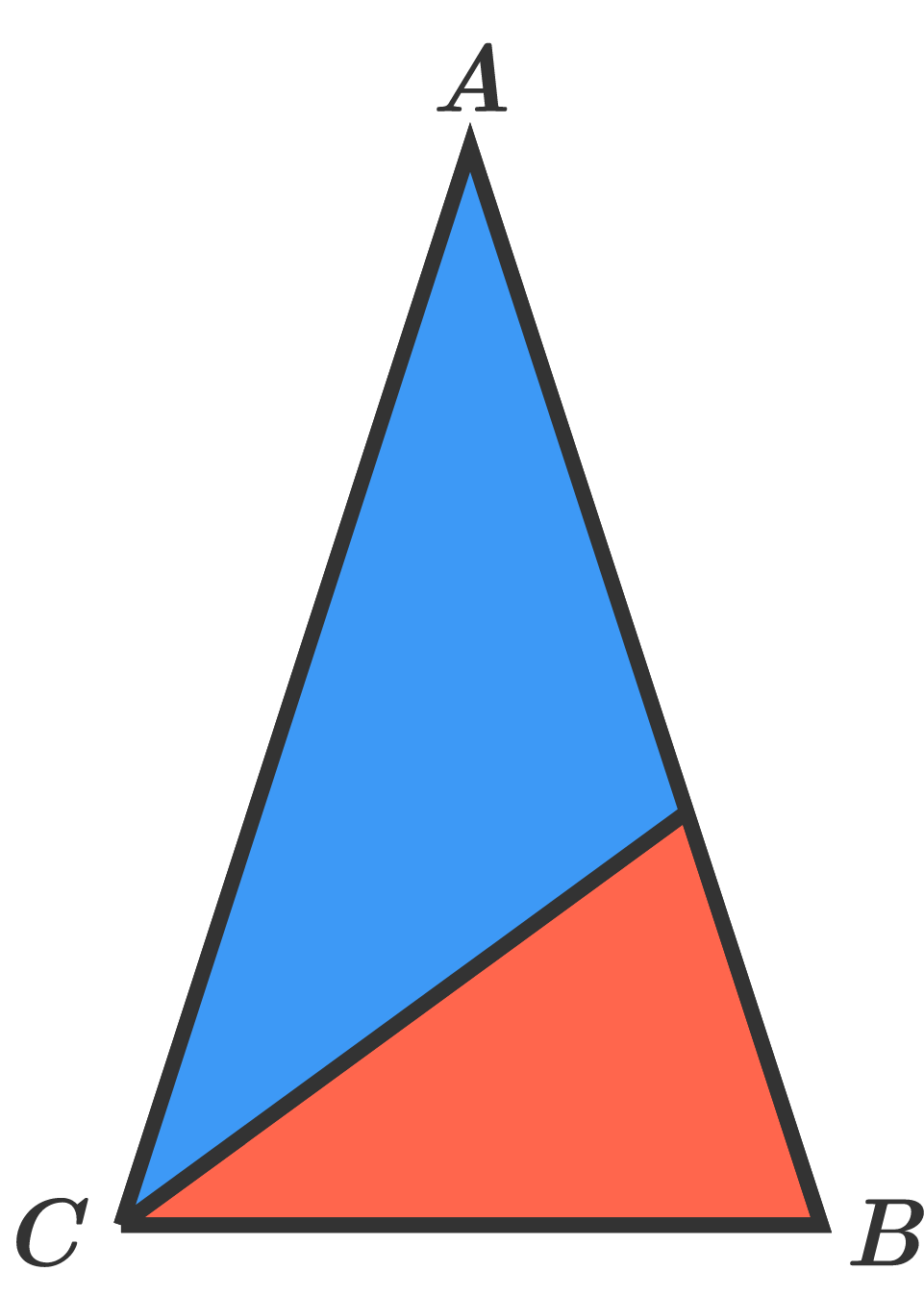# Problems of the Week

Contribute a problem

# 2018-08-20 IntermediateYou're on Space Station Dodecahedron (shaped exactly like a regular dodecahedron), where each vertex is a research node, and the edges are tubes which connect the nodes.

To fix a critical failure in life support systems, you must travel by connecting tubes, visiting each node of the space station exactly once, and then return to your starting node.

Is this possible?

Hint: The vertex graph of a dodecahedron is provided below.A user attempting to solve a problem has only been able to determine that the answer is a 2-digit positive integer.

If the user has 3 tries to guess the answer, what is the approximate chance of getting it correctly?Laurie is standing on the edge of a cliff at a height of $h$ above Orlando. Laurie runs horizontally away from Orlando at constant speed just as he throws a ball towards her. The ball reaches a height of $2h,$ and then Laurie catches the ball as it falls.

What is the ratio of Laurie's speed to the ball's horizontal speed?

Details and Assumptions:

• Treat the ball and each person as points.
• There is downward gravitational acceleration $g.$
• Neglect air resistance.One possible way to draw $ABC$ and split it into two isosceles triangles is shown here.

An isosceles triangle $ABC$ $($with $\angle B=\angle C)$ is cut into two smaller isosceles triangles (which don't have to be identical).

How many different possible angles $\angle A$ are there?

In each of the cases below, there exists only one solution whereby the sum of $n$ positive integers equals the product.

• For $n=2$, we have $2+2=2\times 2$.
• For $n=3$, we have $1+2+3=1\times2\times3$.
• For $n=4$, we have $1+1+2+4=1\times 1\times2\times4$.

If solution(s) exist for $n=5,$ find the sum of all possible sums (or equivalently, products).

If such a solution does not exist, submit your answer as 0.

×